SOLVING MAGNETOSPHERIC ACCELERATION, RECONNECTION AND TURBULENCE

REAL TIME
SPACE WEATHER

 STATUS INDEX 23.87 PCP 1.2 PKP 1.0 KP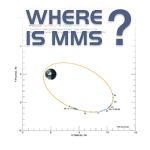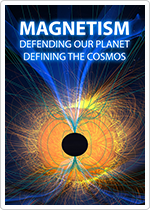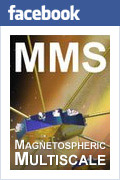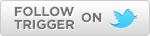Maxwell's Equations PART 1

Maxwell's Equations are probably the most important equations that a space scientist ever has to solve! Just 150 years ago, James Clerk Maxwell took the known equations of electricity and magnetism, and added a single term to one of the equations, and wow - he was able to bring electricity and magnetism together! He then could explain how light works, even in the vacuum of space! Before then, people thought that light needed a medium - the ether - in order for the wave to propagate (move from one place to another), like sound waves need air (or a solid) in order to travel to another place.

But Maxwell showed them that light doesn't need anything at all but changing Electric and Magnetic fields, and that, in a vacuum, light always travels at c (the speed of light), 300,000 kilometers per second. This is 186,000 miles per second, or 670 MILLION miles per hour! (Light wlll slow down in mediums like water or glass... that's how we have lenses, but that's another story).

Now, these equations aren't easy to understand... they require calculus! (Which Newton invented to study Physics, but again that's another story). However, let's see how each of these equations can be understood in terms of CHANGES.

First, though, we need to think about Lines of Force. A force is a push or a pull. We know that a force is a vector: it has a magnitude and a direction. So, the force of gravity on us points from our center of mass to the center of the Earth. That force is our weight, and is a vector, and is measured in Newtons. (Our body mass, which is measured in Kilograms, is a scalar quantity: a scalar has no direction associated with it. So for example, the air temperature is a scalar. The wind is a vector, with speed and direction.).

A line of force then traces out the direction of a force through space. An electric line of force then basically maps the Electric field through space. When we draw sample lines of force, it helps us to understand the strength and direction of the field.

An Electric Field (written as a boldface E) is a force field caused by electrical charges. Imagine a spherical ball with a positive electrical charge on it (maybe it's a glass ball you rubbed on a sweater). The electrical force points away from that ball in all directions. A small positive charge brought near to the ball would feel a force directly away from the ball. So the Electric lines of force start on the ball and go away in all directions (see the left side of the figure, with the yellow positive ball in the center). If the ball had a negative charge (red ball at the right), the electrical lines of force would point towards the ball from all directions: the electric lines of force end on the negative charge, and a small positive charge would be attracted to the ball. The field lines diverge; that is, they get farther apart, the farther you are from the charge, so the electric field gets weaker with distance. Numerically, the field falls off with the square of the distance - if you go from 1 m to 3 m away from the charge, the field strength is 1/9 as strong (1/3, squared). The strength of a field can be thought of as the number of field lines per unit area that they cross. So as the distance from the charge increases from 1 to 3m, the surface area of the sphere enclosing the charge increases from 4π m2 to 36π m2. That is why the field strength goes down as the square of the distance.

Coulomb's Lawis the first Maxwell's equation, which basically just quantifies this effect. If you put a box around a charge, you can tell whether there is positive charge in the box or negative charge in the box based on whether more lines of force are coming out of the box or going into the box. If there are more lines going out than coming in, you have a net positive charge in the box (left), and we call this a positive divergence. If there are more lines coming in, there is net negative charge in the box, so a negative divergence. If instead, the same number of lines come in as go out (right hand side), then there is no net charge in the box. The field has no divergence there. The charge density rho (Greek version ρ) is then just the charge in the box divided by the volume of the box, which can be positive, negative, or zero.

Coulomb's Law uses the divergence operator "Del dot":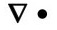that quantifies this spreading. A scientist speaking this equation would say "The divergence of E equals rho over epsilon-naught", or just "Div-E equals ρ over ε-naught". That's why we call this equation "Divvy".

More to come - stay tuned!